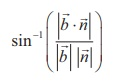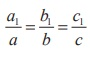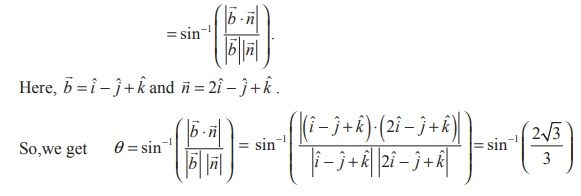Home | | Maths 12th Std | Angle between a line and a plane

# Angle between a line and a plane

(i) If the line is perpendicular to the plane, then the line is parallel to the normal to the plane. (ii) If the line is parallel to the plane, then the line is perpendicular to the normal to the plane.

Angle between a line and a plane

We know that the angle between a line and a plane is the  complement of the angle between the normal to the plane and the lineLet=+ tbe the equation of the line and= p be the equation of the plane. We know thatis parallel to the given line andis normal to the given plane. If θ is the acute angle between the line and the plane, then the acute angle betweenandis ((π/2)-θ).Therefore,So, the acute angle between the line and the plane is given by θ =….(1)

In Cartesian form ifand ax + by + cz = p are the equations of the line and the plane, then= a1iˆ + b1 ˆj + c1kˆ and= aˆi + bˆj + cˆk . Therefore, using (1), the acute angle θ between the line and plane is given byRemark

(i) If the line is perpendicular to the plane, then the line is parallel to the normal to the plane.

So,is perpendicular to. Then we have= λλ R ,which gives(ii) If the line is parallel to the plane, then the line is perpendicular to the normal to the plane.

Therefore,.= 0 aa1 + bb1 + cc1 = 0

Example 6.48

Find the angle between the straight line= (2ˆi + 3ˆj + ˆk )+ ti - ˆj + ˆk ) and the plane 2x - y + z = 5 .

Solution

The angle between a line=+ tand a plane= p with normalis θStudy Material, Lecturing Notes, Assignment, Reference, Wiki description explanation, brief detail
12th Mathematics : UNIT 6 : Applications of Vector Algebra : Angle between a line and a plane |